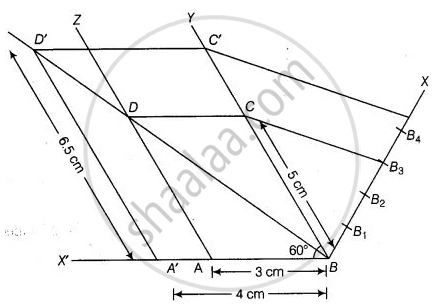# Draw a parallelogram ABCD in which BC = 5 cm, AB = 3 cm and ∠ABC = 60°, divide it into triangles BCD and ABD by the diagonal BD. Construct the triangle BD' - Mathematics

Diagram
Sum

Draw a parallelogram ABCD in which BC = 5 cm, AB = 3 cm and ∠ABC = 60°, divide it into triangles BCD and ABD by the diagonal BD. Construct the triangle BD' C' similar to ∆BDC with scale factor 4/3. Draw the line segment D'A' parallel to DA where A' lies on extended side BA. Is A'BC'D' a parallelogram?

#### Solution

Steps of construction

1. Draw a line segment AB = 3 cm.

2. Now, draw a ray BY making an acute ∠ABY = 60°.

3. With B as centre and radius equal to 5 cm draw an arc cut the point C on

4. Again draw a ray AZ making an acute ∠ZAX’ = 60°. .....[∴ BY || AZ, ∴ ∠YBX’ = TAX’ = 60°]

5. With A as centre and radius equal to 5 cm draw an arc cut the point D on AZ.6. Now, join CD and finally make a parallelogram ABCD

7. Join BD, which is a diagonal of parallelogram ABCD

8. From B draw any ray BX downwards making an acute ∠CBX.

9. Locate 4 points B1, B2, B3, B4 on BX, such that BB1 = B1B2 = B2B3 = B3B4,

10. Join B4C and from B3C draw a line B4C’ || B3C intersecting the extended line segment BC at C’.

11. From point C’ draw C’D’ || CD intersecting the extended line segment BD at D’. Then, AD’BC’ is the required triangle whose sides are  of the corresponding sides of ΔDBC

12. Now draw a line segment D’A’ parallel to DA, where A’ lies on extended side BA i.e ray BX’.

13. Finally, we observe that A’BCD’ is a parallelogram in which A’D’ = 6.5 cm A’B = 4 cm and ∠A’BD’ = 60° divide it into triangles BCD’ and A’BD’ by the diagonal BD.

Concept: Division of a Line Segment
Is there an error in this question or solution?

#### APPEARS IN

NCERT Mathematics Exemplar Class 10
Chapter 10 Construction
Exercise 10.4 | Q 2 | Page 117
Share